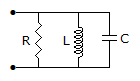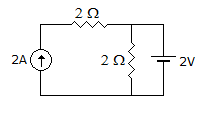# Electronics and Communication Engineering - Networks Analysis and Synthesis

1.

The circuit in figure, is to be scaled to an impedance level of 5kΩ and resonant frequency of 5 x 106 rad/sec. The possible combination of R, L, C isA. 2.5Ω, 0.2 mH, 200 pF B. 5 kΩ, 0.2 mH, 200 pF C. 5 kΩ, 0.2 mH, 200 μF D. 5 kΩ, 0.1 mH, 0.4 μF

Explanation:

If L = 0.2 mH and C = 200 pF, ω0 = 5 x 106 rad/sec and if R is 5 kΩ, impedance level is 5 kΩ.

2.

In figure, the total power consumed isA. 10 W B. 12 W C. 16 W D. 20 W

Explanation:

Use superposition theorem.

3.

If operator 'a' = 1 ∠120° then (1 + a) =

 A. -a B. -a2 C. a D. -1

Explanation:

Since 1 + a + a2 = 0, 1 + a = - a2.

4.

A current wave is i = 100e-60t The initial and final values of current are

 A. 100 and 50 B. 100 and 0 C. 0 and 100 D. 100 and 100

Explanation:

i(0) = 100 e-0 = 100, i(∞) = 100 e-∞ = 0.

5.

Assertion (A): The impedance of a series resonant circuit is minimum at resonance.

Reason (R): Resonance condition implies unity power factor condition.

 A. Both A and R are true and R is correct explanation of A B. Both A and R are true and R is not the correct explanation of A C. A is true but R is false D. A is false but R is true